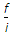# Civil Engineering - Surveying

Exercise : Surveying - Section 3
36.
Pick up the correct statement from the following :
in the earth's magnetic field, a magnetic needle rests in magnetic meridian
the angle between the true meridian and the magnetic meridian is called magnetic variation
one end of the magnetic needle supported at its centre of gravity tends to dip down towards the. nearer magnetic pole of the earth
the magnet properly pivoted is balanced by means of riding weight movable along the needle
all the above.
Explanation:
No answer description is available. Let's discuss.

37.
The formula for the horizontal distances for inclined sights, on staff held normaliss cos θ + (f + d) cos θ ± h sin θ
minus sign is used for angle of depression
plus sign is used for angle of depression
minus sign is used for angle of elevation
non of these.
Explanation:
No answer description is available. Let's discuss.

38.
Ramsden eye-piece consists of
two convex lenses short distance apart
two concave lenses short distance apart
one convex lens and one concave lens short distance apart
two plano-convex lenses short distance apart, with the convex surfaces facing each other.
Explanation:
No answer description is available. Let's discuss.

39.
The distance between steps for measuring down hill to obtain better accuracy
decreases with decrease of slope
increases with increase of slope
decreases with increase of slope
decreases with decrease of weight of the chain.